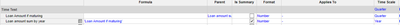# Date input queryHi,

I have a module with various bank loans and maturities e.g. 2020-2028 - this is dimensioned by quarters.

I want to create a module that sums up the total bank loans by maturity.  I've used a formula YEAR to extract the maturity year, but I can't seem to be able to sum on this year?

Can anyone assist?
Thanks!

Tagged:

•Hi @CommunityMember113484 create a line item and capture the quater value if it is maturing then create another line item with time dimension as year and write a formula to pull data from line item where you are storing loan amount if maturing.

You need to enable summary for line item of laon amount if maturing.

Below screenshot for ref.•Hi- thanks for your response. Can you explain how I am able to capture the quarter value of when it is maturing?
In my input module, I have a maturity date and loan value, dimensioned by quarters.
I have now created a new module per your screenshot, but how do I get total loan value by quarter? In my module, my total loan amount is showing in Q3 2020 because that is the value input for Q3 2020, rather than maturing Q3 2020?

•Hi @CommunityMember113484 Write a condition in "Loan Amount if maturing" line item saying if mature year is equal to time scale year then take the mature value else keep zero. Then you will get sum values of loan amount which are maturing that year at year dimension.

•Thanks - what would the formula for time scale year look like?
•@CommunityMember113484  if your target line item dimension is Year than automatically you get the sum if summary is enabled for source line item.

Let us connect and discuss if you still not able to get it.